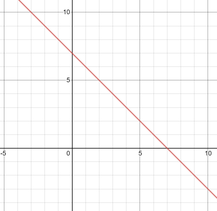# Graphing Functions Worksheets

How to Graph a Function Graphing functions often require making a pattern. A pattern is anything that repeats itself, i.e., a particular action taking place again and again at regular intervals. In this way, a straight line is a pattern where all the points that are connected with each other are formed on the same plane. Suppose you have an equation to graph x + y = 7. The equation means that when the two variables are added, you get 7. They can 1 and 6, 5 and 2, 4 and 3 or 7 and 0. Given that we put these numbers in the form of a table and start plotting them accordingly, we will get a pattern that will be represented in the form of a straight line. Plotting the graph for x + y = 7:The pattern, in this case, is evident as when we join all the points, it forms a straight line.

• ### Basic Lesson

Demonstrates how to graph linear functions. Practice problems are provided. Linear Functions are straight lines defined by the equation: y = mx + b If m is positive, the line slants upwards. As value of m increases the line tends to becomes vertical with extremely large value of m.

• ### Intermediate Lesson

Explores how to graph the absolute value of a function and exponential functions. Practice problems are provided. Absolute Value of function graph takes a V shape and is defined by: y = |x|. As the coefficient of x gets larger, the graph becomes thinner, closer to its line of symmetry.

• ### Independent Practice 1

Contains 20 Graphing Functions problems. The answers can be found below.

• ### Independent Practice 2

As the value of m gets smaller closer to zero the line tends to become horizontal. If m is negative the line slants downhill. By placing any two points of a line into the equation y = mx + b you can determine the equation that it represents.

• ### Homework Worksheet

12 Graphing Functions problems for students to work on at home. Example problems are provided and explained.

• ### Topic Quiz

What type of function is graphed below? A math scoring matrix is included.

• ### Homework and Quiz Answer Key

Answers for the homework and quiz.

• ### Lesson and Practice Answer Key

Answers for both lessons and both practice sheets.

#### Fun Fact:

John Napier's study of mathematics was only a hobby. He often wrote that he found it difficult to find time for the necessary calculations between working and theology.